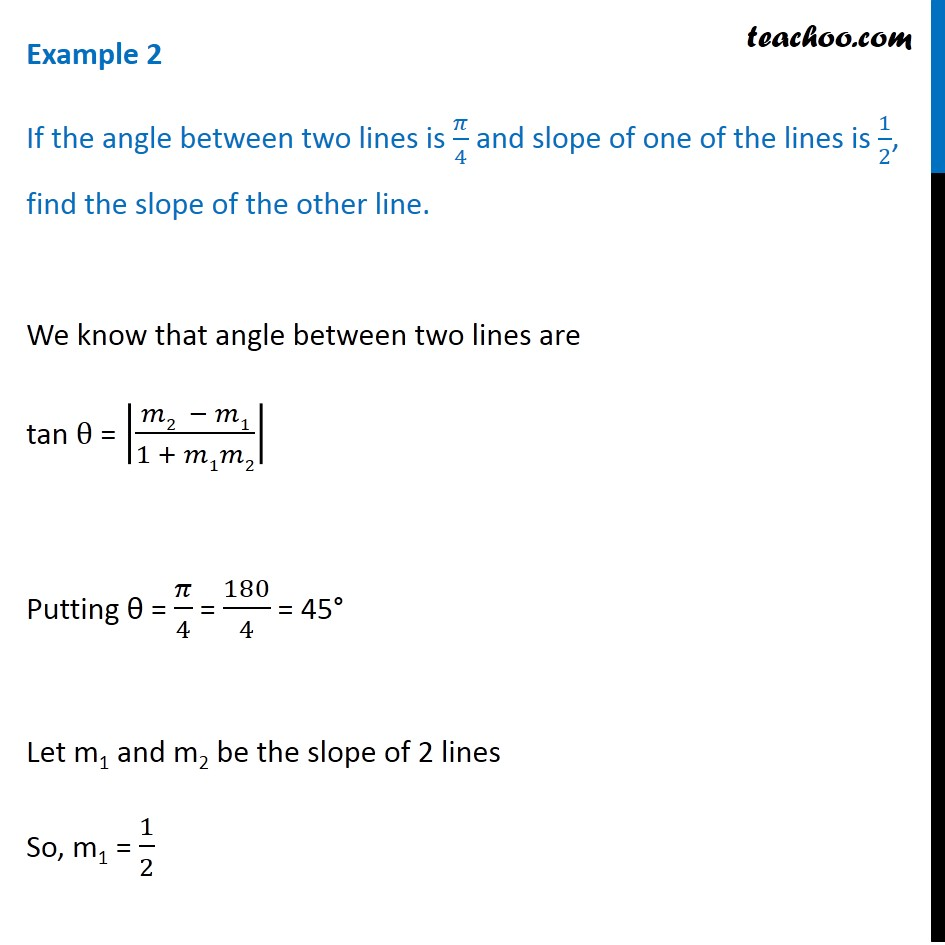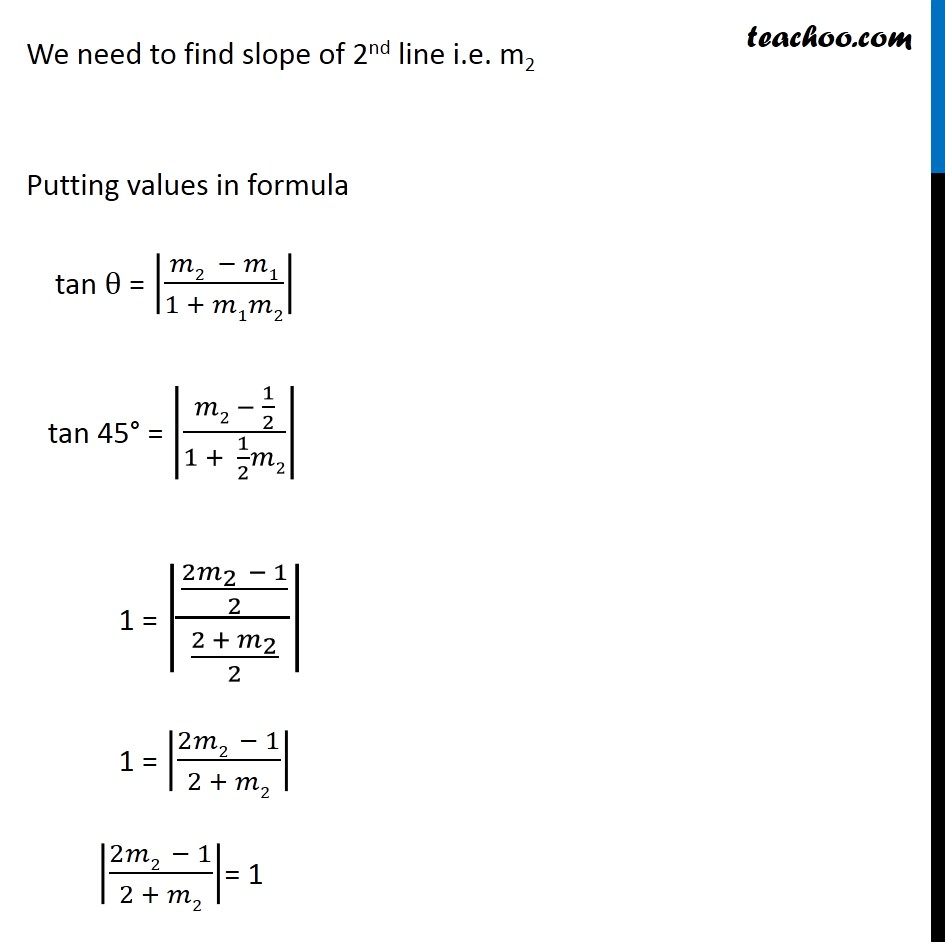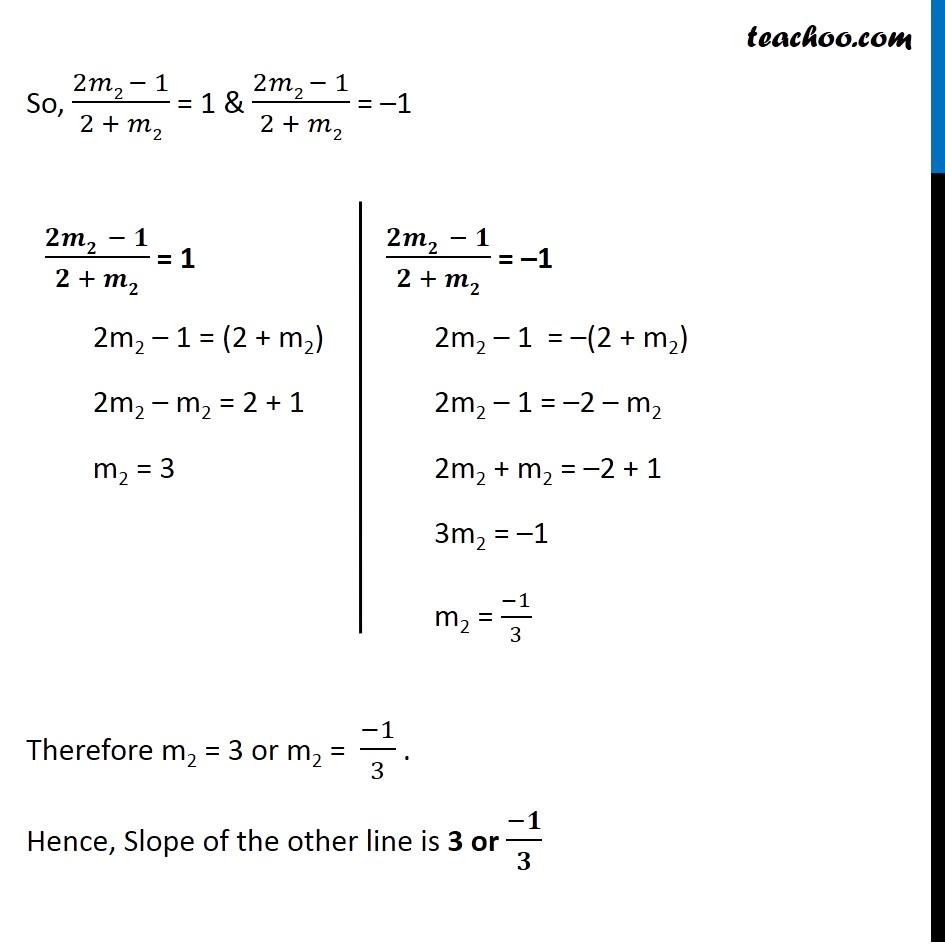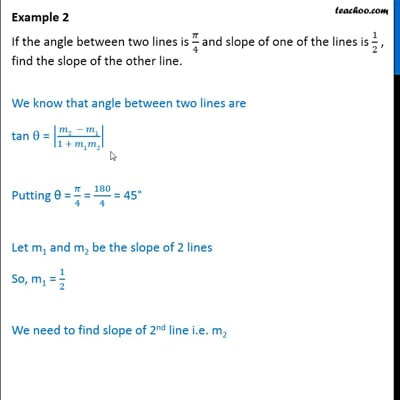Examples

Chapter 10 Class 11 Straight Lines
Serial order wiseThis video is only available for Teachoo black users

Get live Maths 1-on-1 Classs - Class 6 to 12

### Transcript

Example 2 If the angle between two lines is 𝜋/4 and slope of one of the lines is 1/2, find the slope of the other line. We know that angle between two lines are tan θ = |(𝑚2 − 𝑚1)/(1 + 𝑚1𝑚2)| Putting θ = 𝜋/4 = 180/4 = 45° Let m1 and m2 be the slope of 2 lines So, m1 = 1/2 We need to find slope of 2nd line i.e. m2 Putting values in formula tan θ = |(𝑚2 − 𝑚1)/(1 + 𝑚1𝑚2)| tan 45° = |(𝑚2 − 1/2)/(1 + 1/2 𝑚2)| 1 = |((2𝑚_2 − 1)/2)/((2 + 𝑚_2)/2)| 1 = |(2𝑚2 − 1)/(2 + 𝑚2)| |(2𝑚2 − 1)/(2 + 𝑚2)|= 1 So, (2𝑚2 − 1)/(2 + 𝑚2) = 1 & (2𝑚2 − 1)/(2 + 𝑚2) = –1 Therefore m2 = 3 or m2 = (−1)/3 . Hence, Slope of the other line is 3 or (−𝟏)/𝟑 (𝟐𝒎𝟐 − 𝟏)/(𝟐 + 𝒎𝟐) = 1 2m2 – 1 = (2 + m2) 2m2 – m2 = 2 + 1 m2 = 3 (𝟐𝒎𝟐 − 𝟏)/(𝟐 + 𝒎𝟐) = –1 2m2 – 1 = –(2 + m2) 2m2 – 1 = –2 – m2 2m2 + m2 = –2 + 1 3m2 = –1 m2 = (−1)/3